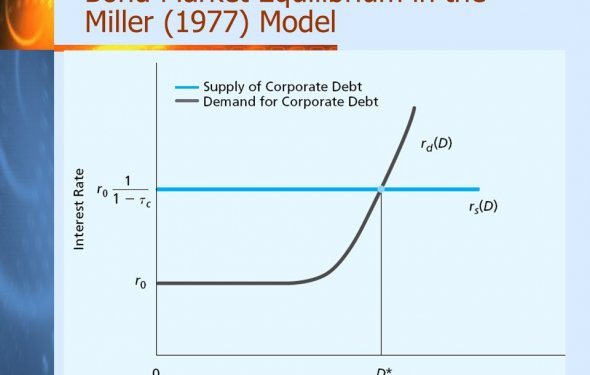# Bond market equilibrium

The Supply and Demand for Bonds
June 25, 2017 – 11:08 am### Figure 2. Supply function for bonds

Demand and supply functions intersect at one point, the equilibrium. Equilibrium reflects a state of rest. As long as the supply or demand function does not change, then the price and quantity remain where they are. We show supply and demand functions in Figure 3. At this point, the quantity demanded equals the quantity supplied for bonds. The Q* and P* represent equilibrium quantity and price. Using the present value formula, we can deduce what happens tothe market interest rate.

What would happen to the bond market if the bond's price exceeds the equilibrium price? Consequently, the quantity supplied is greater than quantity demanded, creating a surplus. Businesses and government sell more bonds because the price of bonds is high, and interest rates are low. However, the investors do not buy these bonds because the high price and lowinterest rates. Thus, the bond's price falls until restoring equilibrium at P* again.

### Figure 3. Supply and demand for bonds

What would happen in the market if the price of bonds were lower than the equilibrium price? Quantity supplied becomes less than quantity demanded, creating a shortage. Bond prices are low, and interest rates are high. Consequently, the investors have a large demand for bonds because the bonds make a good investment. However, businesses and government do not sellbonds for a low price and high interest rate. Thus, the bond's price must increase until equilibrium is restored at P* again, decreasing the market interest rates. Therefore, the market always gravitates to equilibrium and consistently eliminates shortages and surpluses as long as a government does not interfere in the market.

Demand function can shift because a factor has changed. Please know the difference between a movement along a demand curve and a demand function shift. We show a decrease in quantity demanded in Figure 4. Investors demand more bonds as we move from point A to point B. Economists call this a change in "quantity demanded. " Investors increase quantity demanded because the price of bonds became cheaper. Consequently, a factor has changed the supplyfunction and not the demand function. If an outside factor affects the demand function, then the demand function would shift. Economists call a rightward shift an "increase in demand, " while a shift to the left is a "decrease in demand. " We show demand function shifts in Figure 5.

### Figure 5. A demand function shifts

We listed six factors to show an increase in the demand function, shifting it rightward. We show the increase in the demand function in Figure 6. When the investors increase their demand for bonds, the demand function shifts rightward because investors buy more bonds. Thus, both the equilibrium quantity, Q*, and bond's market price, P*, rise. When we discount the bondsusing the present value formula, the market interest rate for the bonds fall.

Source: www.boundless.com
Related Posts